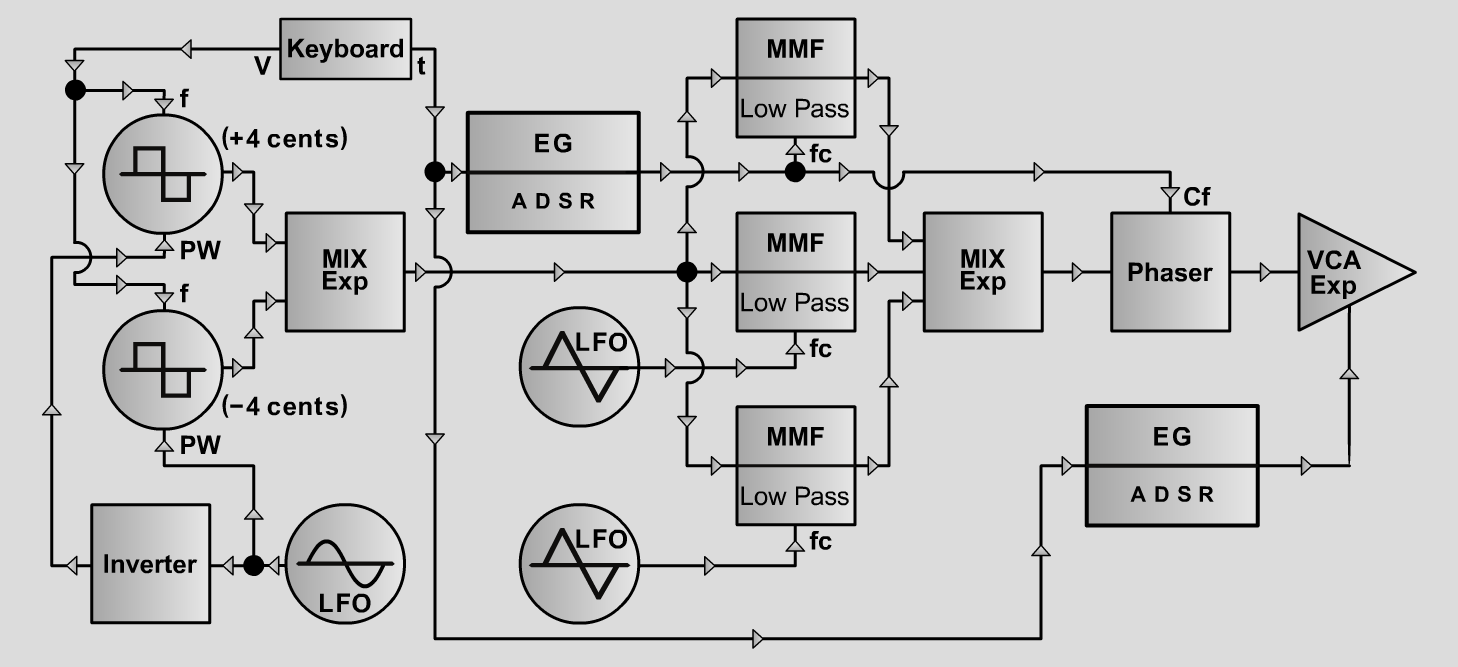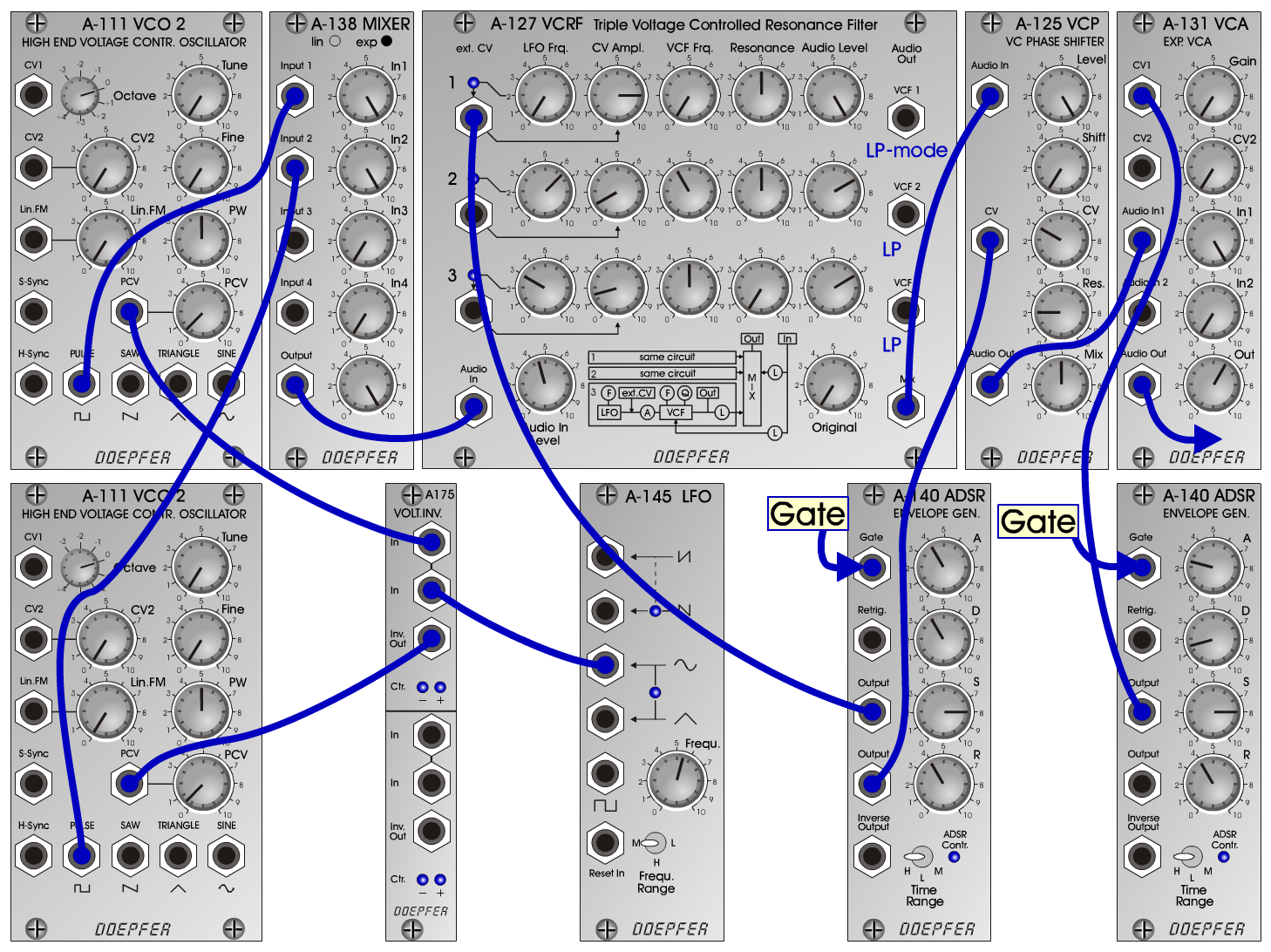Birth of PlanetS y n t h- P a dD o e p f e r A - 100 Connections: Settings: A-111/1 (Pulse) <=> A-138exp (Input 1)  A-111/2 (Pulse) <=> A-138exp (Input 2)  A-138exp (Output) <=> A-127 (Audio In)  A-127 (Mix) <=> A-125 (Audio In)  A-125 (Audio Out) <=> A-131 (Audio In)  A-145 (Sine) <=> A-175 (In)  A-175 (In/2) <=> A-111/1 (PCV)  A-175 (Inv Out) <=> A-111/2 (PCV)  A-140/1 (Output) <=> A-131 (CV1)  A-140/2 (Output/1) <=> A-127 (ext. CV 1)  A-140/2 (Output/2) <=> A-125 (CV) A-111/1 (PW = 5, PCV = 0.5)  A-111/2 (PW = 5, PCV = 0.5)  A-127/LP-mode (Audio In = 4.5, Original = 0;    Row 1: LFO Freq = 0, CV Ampl = 8,    VCF Frq = 0, Res = 5, Audio Level = 10;    Row 2: LFO Freq = 6.5, CV Ampl = 1,    VCF Frq = 4, Res = 5, Audio Level = 7;    Row 3: LFO Freq = 3, CV Ampl = 1.5,    VCF Frq = 5, Res = 0, Audio Level = 7)  A-125 (Level = 10, Shift = 0, CV = 3, Res = 2, Mix = 5)  A-145 (Frq = 5.5, Range = M)  A-140/1 (A = 2.5, D = 1.5, S = 8, R = 4, Range = H)  A-140/2 (A = 4, D = 4, S = 8, R = 4, Range = H)  A-138exp (In 1 = 10, In 2 = 10, Out = 10)  A-131 (Gain = 0, Audio In 1 = 10, Audio Out = 6) Tuning: A-111/1 => +4 cents; A-111/2 => -4 cents; Josef MuellerSound samples Birth of Planet Piece Birth of Planet A Birth of Planet B Birth of Planet C
br>